# The Magnetic Tower of Hanoi

 Page 7/20 Date 23.04.2018 Size 2.78 Mb. #45758

Table 4: Minimum number of disk-moves required to solve the Colored Magnetic Tower of Hanoi puzzle. N is the total number of disks participating in the game and k is the disk number in the ordered stack, counting from bottom to top. The k-th disk "makes" 3(k-1) moves (Equation 7). The total number of disk-moves required to solve an N-disk puzzle is (3N – 1)/2 (Equation 8).
Having solved the rather simple Colored Magnetic Tower puzzle, we can move on to solving the more intricate "Free" or "Dynamically Colored" Magnetic Tower puzzle. As discussed below, we will identify "Free" and "Colored" states of the "Dynamically Colored" MToH leading to a far "shorter" solution (relative to the "100" solution) of the MToH puzzle.

1. The "67%" solution of the MToH puzzle

The color of posts in the MToH puzzle is determined by the color of the disks it holds. The color is therefore "dynamic". During the game, the color of a given post can be RED, can be BLUE, and can be Neutral. For moves analysis, we can distinguish between three distinct MToH states.

1. Distinct states of the Magnetic Tower of Hanoi

After playing with the MToH puzzle for a while, one may realize that actually three distinct tower states exist

• "Free" - two posts are Neutral ("start" and "end" states)

• "Semi-Free" – one post Neutral, the other two are oppositely Colored

• "Colored" – two posts are co-colored

During the "game", the tower is some-times "Semi-Free". Which opens the room for significant "savings".

The "67" solution indeed takes advantage of the Tower's occasional "semi-freedom". In fact, as I will show below, for large N, the number of moves for this "67" solution is 2/3 of the number of moves of the "100" solution.

1. The 67% solution

The "67" (percent) solution is based on the sequence listed in Table 5:

 Step # Disks From To # of moves Comments 1 2 to N S (Red) I (Blue) S67(N-1) "Free" MToH 2 1 S (Red) D (Blue) 1 3 3 to N I (Blue) D (Blue) 2*S100(N-2) Through "Red" S 4 2 I (Blue) S (Red) 1 5 3 to N D (Blue) I (RED) 1*S100(N-2) 6 2 S (Red) D (Blue) 1 7 3 to N I (RED) D (Blue) 1*S100(N-2) Puzzle solved

Table 5: The sequence of moves for the "67" solution of the MToH puzzle.
As shown, the total number of moves is
S
67(N) = S67(N-1) + 4*S100(N-2) + 3.

If we start on a Red post (up-facing surfaces of all disks are colored Red), then after S67(N-1) + 1 moves we arrive at the state described by Figure 7. The rest of the move-sequence to solve the puzzle is 4*S100(N-2) + 2, as detailed in the text and as listed in Table 5.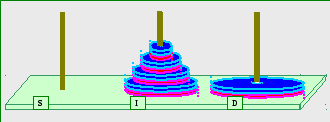Figure 7: Moving N-disks from S to D by the "67" Algorithm. The figure shows state of the tower (started Red on Post S) after N-1 disks are moved to the Intermediate Post and the N-th disk is moved to the Destination Post. The number of (minimum) moves to get from puzzle-start state to the figure-described state is S67(N-1) + 1. The rest of the move-sequence to solve the puzzle is 4*S100(N-2) + 2, as detailed in the text and as listed in Table 5.
The recursive proof of the "67" Algorithm is the following: we know how to solve for 3 disks. For N > 3, if the algorithm works for N disks, it works for N+1 disks because after we have successfully moved N disks ("down") from S to I (as assumed) and moved the N+1 disk from S to D in a legal way (Figure 7), we move N-2 disks using the always legal "Colored" algorithm (steps 3, 5, 7 in Table 5) and move the N-1 single disk twice in a legal way (steps 4 and 6 in Table 5).

The number of moves in the "67" solution Algorithm as explained above is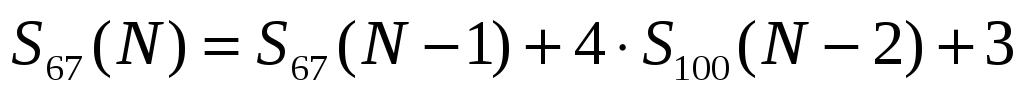. (9)
Given Equation 7 and Equation 9, we can quickly formulate non-recursive expressions to the number of moves. Consulting these two equations and performing some algebric manipulations, we find for the "67" solution of the Magnetic Tower of Hanoi –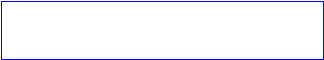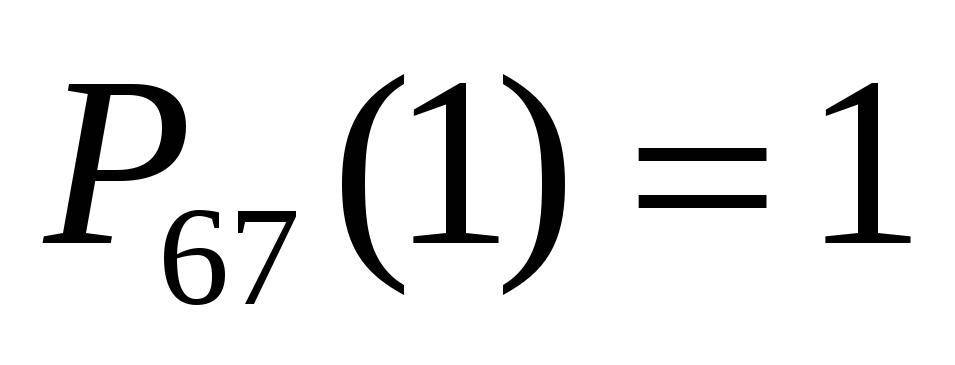(10)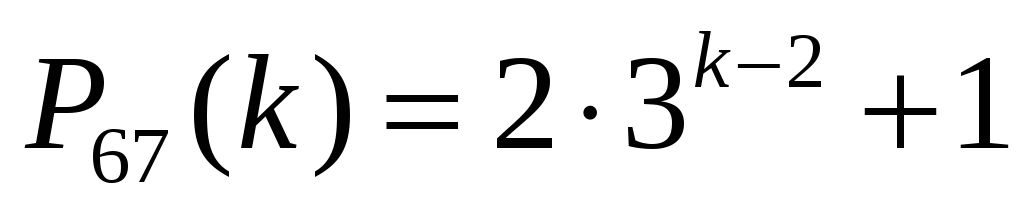;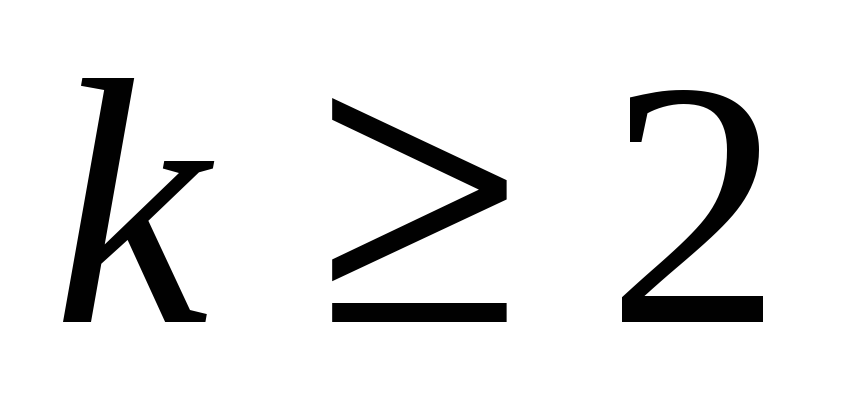And from Equation 10: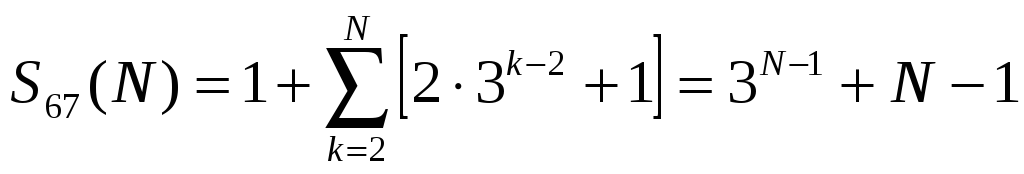. (11)
Just copying Equation 11 for clarity: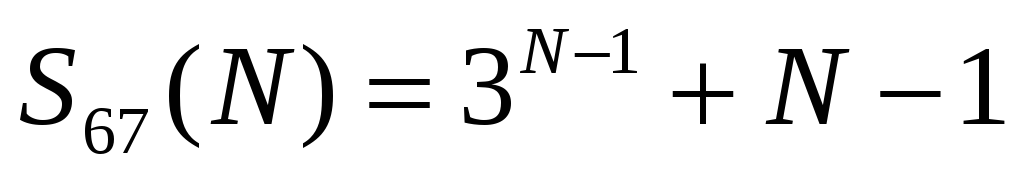. (12)
Table 6 lists the number of moves of each disk (Equation 10) and the total number of moves (Equation 12) for the "67" solution of the MToH puzzle, for the first eight stack heights.